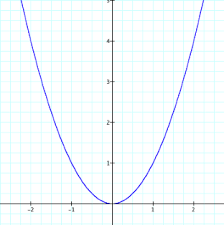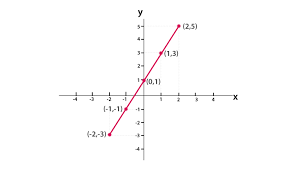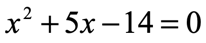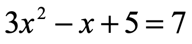Math Rules
Linear Functions
Linear Graphs
Math Riddles
100

What is the slope in the equation: y=189x+22

m = 189

100

Find the answer by interpreting the statement: If f(x)=31x-12 and x=2

f(2)=50

100

Which lines have a positive slope?Graphs I, II, and III

100

What weighs more, a pound of iron or a pound of feathers?

They both weigh the same.

100

What is the standard form of the quadratic equation?

ax2+bx+c=0

200

What is the y-intercept for the function: g(x)=−78+5x

b = -78

200

Find the answer by interpreting the statement: If f(x)=-100x2-50 and x=10

f(10)=-10,050

200

What is the equation of this graph?y = 2x+8

200

If you multiply this number by any other number, the answer will always be the same. What number is this?

Zero

200300

How do you find the area of a triangle?

Area of a Triangle = (1/2)x(base)x(height)

300

What is the input value for the function f(x)=−7x+81 when x = 6000

6000

300

What is the y-intercept of this graph?4

300

I add five to nine and get two. The answer is correct, but how?

When it is 9 p.m., add 5 hours to it and you will get 2 p.m.

300

What is the equation of this graph?y=x2

400

How do you find the slope of a linear graph? (You have to say the name of the rule and explain the process)

Rise-over-run: Pick two points on a graph. Rise (up or down) from one point and then run (left or right) to the other point.

400

Find the answer by interpreting the statement: If f(x)=76x−17 and x=3

f(3) = 211

400

What is the equation of this line?y = -2x+1

400

You are given 3 positive numbers. You can add these numbers and multiply them together. The result you get will be the same. Which are the numbers?

1, 2, and 3

400

Solve for x:x1 = 2

x2 = 7

500

How do you find the equation of a linear graph?

Find the slope using rise-over-run. Find the y-intercept by finding a point where the line crosses the y-axis. Then put both numbers in y=mx+b format.

500

Find the answer by interpreting the statement: f(x) = 9x2+10 when x = 7

f(3) = 451

500

What is the equation of this line?y=-(2)/(3)x+5

500

What is the next number in the series? 7,645 5,764 4,576

6,457 because the last number is moved to the front to make the next number in the series.

500

Solve for X:x1 = 1

x2 = -2/3

Click to zoom